# US20040189243A1 - Apparatus for controlling an a. c. motor - Google Patents

## Info

Publication number
US20040189243A1
US20040189243A1 US10/809,530 US80953004A US2004189243A1 US 20040189243 A1 US20040189243 A1 US 20040189243A1 US 80953004 A US80953004 A US 80953004A US 2004189243 A1 US2004189243 A1 US 2004189243A1
Authority
US
United States
Prior art keywords
motor
axis
current
value
instruction
Prior art date
Legal status (The legal status is an assumption and is not a legal conclusion. Google has not performed a legal analysis and makes no representation as to the accuracy of the status listed.)
Granted
Application number
US10/809,530
Other versions
US7075266B2 (en
Inventor
Kazuaki Tobari
Tsunehiro Endou
Yoshitaka Iwaji
Hidefumi Shirahama
Yasuo Notohara
Current Assignee (The listed assignees may be inaccurate. Google has not performed a legal analysis and makes no representation or warranty as to the accuracy of the list.)
Hitachi Ltd
Original Assignee
Hitachi Ltd
Priority date (The priority date is an assumption and is not a legal conclusion. Google has not performed a legal analysis and makes no representation as to the accuracy of the date listed.)
Filing date
Publication date
Priority to JP2003089571A priority Critical patent/JP3832443B2/en
Priority to JP2003-089571 priority
Priority to JP2003198838A priority patent/JP2005039912A/en
Priority to JP2003-198838 priority
Application filed by Hitachi Ltd filed Critical Hitachi Ltd
Assigned to HITACHI LTD. reassignment HITACHI LTD. ASSIGNMENT OF ASSIGNORS INTEREST (SEE DOCUMENT FOR DETAILS). Assignors: SHIRAHAMA, HIDEFUMI, TOBARI, KAZUAKI, IWAJI, YOSHITAKA, ENDOU, TSUNEHIRO, NOTOHARA, YASUO
Publication of US20040189243A1 publication Critical patent/US20040189243A1/en
Application granted granted Critical
Publication of US7075266B2 publication Critical patent/US7075266B2/en
Active legal-status Critical Current

• 230000001276 controlling effects Effects 0.000 title claims description 44
• 244000171263 Ribes grossularia Species 0.000 claims abstract description 262
• 230000000694 effects Effects 0.000 description 14
• 235000020127 ayran Nutrition 0.000 description 12
• 230000001131 transforming Effects 0.000 description 9
• 230000004907 flux Effects 0.000 description 4
• 230000005355 Hall effect Effects 0.000 description 3
• 230000000875 corresponding Effects 0.000 description 3
• 230000035832 Lag time Effects 0.000 description 2
• 230000035648 Lag-time Effects 0.000 description 2
• 229920000535 Tan II Polymers 0.000 description 2
• 239000011159 matrix materials Substances 0.000 description 2
• 230000004048 modification Effects 0.000 description 2
• 238000006011 modification reactions Methods 0.000 description 2
• 239000007787 solids Substances 0.000 description 2
• 239000003990 capacitor Substances 0.000 description 1
• 238000006243 chemical reactions Methods 0.000 description 1
• 230000020169 heat generation Effects 0.000 description 1
• 238000010438 heat treatment Methods 0.000 description 1
• 238000004519 manufacturing process Methods 0.000 description 1
• 238000005070 sampling Methods 0.000 description 1
• 238000005406 washing Methods 0.000 description 1
• 238000004804 winding Methods 0.000 description 1

## Images

•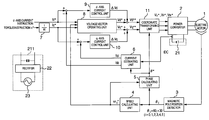•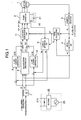•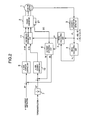•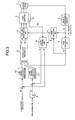•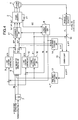•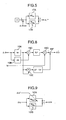•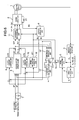•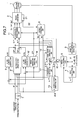••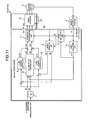••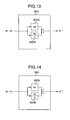•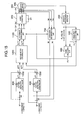••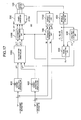•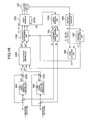•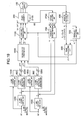•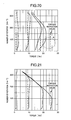•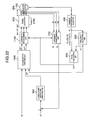## Classifications

• HELECTRICITY
• H02GENERATION; CONVERSION OR DISTRIBUTION OF ELECTRIC POWER
• H02PCONTROL OR REGULATION OF ELECTRIC MOTORS, ELECTRIC GENERATORS OR DYNAMO-ELECTRIC CONVERTERS; CONTROLLING TRANSFORMERS, REACTORS OR CHOKE COILS
• H02P21/00Arrangements or methods for the control of electric machines by vector control, e.g. by control of field orientation
• H02P21/06Rotor flux based control involving the use of rotor position or rotor speed sensors

## Abstract

A method controls a torque of an a. c. motor which does not cause a shortage of torque by using an economical current detection which estimates d- and q-axis currents Idc, Iqc from d. c. current IDC flowing through an input d. c. bus line of a power converter. A value of d- and q-axis motor currents Id, Iq of a rotational coordinate system are estimated from detected input d. c. current IDC flowing through the bus line of the power converter to which power is input from a d. c. power source 21. A output voltages of the power converter 2 are controlled so that the estimated currents Idc, Iqc are equal to respective current instruction values Id*, Iq*. Errors of motor constants are determined from information on the motor currents and the rotational phase errors by an operation.

## Description

BACKGROUND OF THE INVENTION
• The present invention relates to an apparatus for controlling an a. c. motor and a module using the same. 
• A study paper “Development of full automatic washing machine which is controlled by an inverter” reported in research meeting at Ibaraki Office of Tokyo Branch, The Institute of Electrical Engineers of Japan (IEEJ) (1999) describes “an open loop type vector control scheme” is utilized in an electric motor current sensorless, low resolution position detector. 
• Another prior art using a magnetic pole position detector and an electric motor current sensor is disclosed in JP-A-2000-324881 which teaches a control device. In this device an electric current detector directly detects currents flowing through windings of a motor for generating such a voltage instruction that an instructed current is equal to detected currents in a rotary coordinate system. 
• SUMMARY OF THE INVENTION
• It is an object of the present invention to provide an apparatus for controlling an a. c. motor which does not cause a shortage of torque in a low speed range without being influenced by variations in constants of the motor and mounting errors of a Hall effect element and the like. 
• One of the features of the present invention resides in that motor currents Id, Iq on the d- and q-axes of a rotary coordinate system are estimated and the voltage output from a power converter are controlled so that the estimated currents Idc, Iqc are equal to respective current instruction values Id*, Iq*. 
• Another feature of the present invention resides in that an apparatus for controlling an a. c. electric motor comprises current estimating means which receives detected input d. c. currents from a power converter for converting d. c. power into a. c. power and the rotational phase which is obtained from a signal of detected position of the a. c. motor for outputting estimated current values of the a. c. motor on the d- and q-axes of the rotational coordinate system of the motor, d-axis current controlling means for controlling the d-axis current so that said estimated current value approaches the d-axis current instruction value, and q-axis current controlling means for controlling the q-axis current so that said estimated current value approaches the q-axis current instruction value. 
• Other objects, features and advantages of the invention will become apparent from the following description of the embodiments of the invention taken in conjunction with the accompanying drawings.
• BRIEF DESCRIPTION OF THE DRAWINGS
• FIG. 1 shows the configuration of a torque control circuit of a permanent magnet synchronization motor of one embodiment of the present invention; 
• FIG. 2 shows the configuration of a torque control circuit of a permanent magnet synchronization motor of another embodiment of the present invention; 
• FIG. 3 shows the configuration of a torque control circuit of a permanent magnet synchronization motor of a further embodiment of the present invention; 
• FIG. 4 shows the configuration of a torque control circuit of a permanent magnet synchronization motor of a further embodiment of the present invention; 
• FIG. 5 shows a frequency operating unit in the apparatus of FIG. 4; 
• FIG. 6 shows the configuration of a torque control circuit of a permanent magnet synchronization motor of a further embodiment of the present invention; 
• FIG. 7 shows the configuration of a torque control circuit of a permanent magnet synchronization motor of a further embodiment of the present invention; 
• FIG. 8 shows a q-axis inductance operating unit in the apparatus of FIG. 7; 
• FIG. 9 shows a q-axis current control unit in the apparatus of FIG. 7; 
• FIG. 10 shows the configuration of the torque control circuit of the permanent magnet synchronization motor of a further embodiment of the present invention; 
• FIG. 11 shows an example to which the present invention is applied; 
• FIG. 12 shows an apparatus for controlling the permanent magnet synchronization motor of one embodiment of the present invention; 
• FIG. 13 shows a d-axis current instruction operating unit  8 in the control apparatus of FIG. 1;
• FIG. 14 shows a q-axis current instruction operating unit  9 in the control apparatus of FIG. 1;
• FIG. 15 shows an apparatus for controlling the permanent magnet synchronization motor of a further embodiment of the present invention; 
• FIG. 16 shows an apparatus for controlling the permanent magnet synchronization motor of a further embodiment of the present invention; 
• FIG. 17 shows an apparatus for controlling the permanent magnet synchronization motor of a further embodiment of the present invention; 
• FIG. 18 shows an apparatus for controlling the permanent magnet synchronization motor of a further embodiment of the present invention; 
• FIG. 19 shows an apparatus for controlling the permanent magnet synchronization motor of a further embodiment of the present invention; 
• FIG. 20 shows the relationship between the number of rotations and the measured torque when the present invention is not used. 
• FIG. 21 shows the relationship between the number of rotations and the measured torque when the present invention is used. 
• FIG. 22 shows an apparatus for controlling the permanent magnet synchronization motor of a further embodiment of the present invention.
• DESCRIPTION OF THE EMBODIMENTS
• Now, the present invention will be described by way of embodiments with reference to annexed drawings. 
• First Embodiment
• Referring now to FIG. 1, there is shown the configuration of an apparatus for controlling a permanent magnet synchronization electric motor which is an embodiment of the present invention. The apparatus comprises a power converter to which a power from a d. c. power source  21 is input, for outputting voltages which are proportional to three-phase a. c. voltage instruction values Vu*−Vw* to an permanent synchronization electric motor 1; a magnetic pole position detector 3 which is capable of detecting the position value θi at every electrical angle 60° of the permanent magnet synchronization electric motor 1; a speed calculating unit 4 for calculating the rotational speed ω1* of the motor 1 from the detected position value θi; a phase calculating unit 5 for calculating the rotational phase θ* of the motor 1 from the detected position value θi and the rotational speed ω1*; a current estimating unit 6 for calculating estimated current values Idc, Iqc on d-axis (corresponding to magnetic flux axis) and q-axis (corresponding to torque axis) of the rotational coordinate system from input d. c. bus current detected value IDC from the power converter 2; a conversion coefficient which is used for calculating the q-axis current instruction value Iq* from a torque instruction value τ*; a voltage vector operating unit 8 for operating voltage reference values Vd* and Vq* based upon constants of the motor, current instruction values Id* and Iq*, and the rotational speed ω1*; a d-axis current control unit 9 for outputting ΔVd as a function of the difference between the d-axis current instruction value Idc; a q-axis current control unit 10 for outputting ΔVq as a function of the difference between the q-axis current instruction value Iq* and the estimated q-axis current value Iqc; and a coordinate transforming unit 11 for outputting the voltage instruction values Vu*−Vw* of three-phase a. c. from the voltage reference values Vd*, Vq*, current control outputs ΔVd, ΔVq and the rotational phase θ* as shown in FIG. 1.
• The d. c. power source  21 may be primary or secondary battery, or may be power from a capacitor or battery which is charged with a power obtained by rectifying commercial power or a. c. power output from a generator 23 as is done in a d. c. power source 211. Description of the d. c. power source will be omitted in the embodiments which will be described below since the d. c. power source can be formed similarly to the foregoing.
• The torque instruction value τ* and the d-axis current instruction value Id* are determined by a host apparatus. For example, the torque instruction value T* is determined depending upon the operation of input devices. The same will be applied to the embodiments which will be described. 
• Components  1 to 5, 7 and 11 are configured similarly to those for the open loop type vector control used in the low resolution position detector which is disclosed as speed control type in the cited prior art.
• Firstly, the basic operation in which the open loop type vector control is applied to the torque control apparatus will be described. 
• In order to control the motor currents Iq, Id depending upon the q-axis current instruction value Iq* and the d-axis current instruction value Id* determined by the torque instruction value τ*, the d- and q-axis voltage reference values Vd* and Vq* are preliminarily calculated in the voltage vector operating unit  8 in accordance with equation (1) for controlling the output voltages from the converter. $( Vd ** = R 1 * · Id ** - ω 1 * · Lq * · Iq ** Vq ** = R 1 * · Iq ** + ω 1 * · Ld * · Id ** + ω 1 * · Ke * ) ( 1 )$
• wherein R1* denotes a preset resistance value, Ld* and Lq* denote preset values of d- and q-axis inductances, Ke* denotes a preset value of induced voltage constant, ω 1* denotes the rotational speed.
• The position of the magnetic pole at every 60° of the electric angle can be determined by the magnetic pole position detector  3. The detected position value θi at this time can be expressed in the present embodiment as follows:
• θi=60i+30   (2)
• wherein i=0, 1, 2, 3, 4, 5. 
• The averaged rotational speed ω 1* over a period of at least 60° can be calculated from the detected position value θi in the speed calculating unit 4 as follows:
• ω1 =Δθ/Δt   (3)
• wherein Δθ is θi−θ(i−1), Δt denotes the time which is taken to detect a position detection signal for a period of 60 degrees. However, due to the presence of mounting errors of the magnetic pole position detector the speed which is averaged over a period of 120° or more has been practically used. 
• The phase calculating unit  5 calculates the rotational phase θ* in accordance with the equation (4) using the detected position value θi and the rotational speed ω1* for controlling the reference phase of the motor 1.
• θ*=θi+ωi*Δt   (4)
• The basic configuration of the voltage control and the phase control in the open loop type vector control scheme has been described. 
• When a high torque is required during torque control operation, it is necessary to cause a high current corresponding to the torque to flow. When a high torque is required for an extended period of time, the resistance value R of the windings in the motor increases with the lapse of time due to heating of the motor caused by the current flowing through the motor. Since the preset resistance value R* which is calculated by the voltage vector operating unit  8 is not equal to the actual resistance value R, the motor 1 can not be supplied with a necessary voltage. As a result, a current which is necessary to generate a requisite torque can not flow, which leads to a shortage of the torque.
• Hence, in the present embodiment, the currents Idc and Iqc of the d- and q-axes of the rotational coordinate system are estimated from the d. c. current IDC flowing through the input current bus line of the power converter. The signals ΔVd and ΔVq which depend on the current deviation are determined by the d- and q-axis current control units  9 and 10, respectively so that the estimated signals are equal to respective instruction values. The voltage output from the converter is changed by adding the signals ΔVd and ΔVq to the outputs of the voltage vector operating unit 8. As a result, even if the R* which is preset by the voltage vector operating unit 8 is not equal to the actual resistance value R, the output voltage can be controlled in such a manner that the currents in the motor are equal to the current instruction values. Thus, high precision torque control can be achieved with a simple configuration without causing a shortage of torque.
• Although the voltage reference values Vd* and Vq* are calculated using instructed current values Id* and Iq* in the voltage vector operating unit  8, respectively in the present embodiment, similar advantage can be provided by using Idc and Iqc which are estimated from the d. c. current IDC.
• Second Embodiment
• Referring now to FIG. 2, there is shown another embodiment of the present invention, which is an apparatus for controlling the torque of the permanent magnetic synchronization motor in which the voltages output from the converter are controlled by controlling only the currents on the d- and q-axis without conducting the operation of the output voltage vector. 
• Components in FIG. 2 which are identical with those in FIG. 1 are represented by reference numerals  1 through 7, 9 through 11, and 21. The difference between the embodiments in FIGS. 1 and 2 resides in that the voltage vector operating unit 8 is omitted. Even if the voltage vector operating unit 8 is omitted, the voltages output from the converter can be controlled by the current control units 9 and 10 in such a manner that Idc and Iqc are equal to respective instructing values. Thus, high precision torque control can be achieved with a simple configuration without causing a shortage of torque.
• Third Embodiment
• Referring now to FIG. 3, there is shown a further embodiment of the present invention, which is an apparatus for controlling the torque of a permanent magnet synchronization motor of the type in which the instruction values Id** and Iq** are obtained from the outputs of the d- and q-axis current instruction calculating units  12, 13. Components in FIG. 3 which are identical with those in FIG. 1 are designated with reference numerals 1 to 7, 11 and 21. A reference numeral 8′ denotes a voltage vector operating unit for operating voltage reference values Vd*** and Vq*** based upon constants of the motor, signals Id** and Iq**, respectively and the rotational speed ω1*. A reference numeral 12 denotes a d-axis current instruction calculating unit for outputting the Id** as a function of the deviation between Id* and Idc. A reference numeral 13 denotes a q-axis current instruction operating unit for outputting Iq** as a function of the difference between Iq* and Iqc. The output voltages of the converter are controlled by calculating the voltage reference values Vd*** and Vq*** represented in the equation (5) using the signals Id** and Iq**, respectively. $( Vd ** = R 1 * · Id ** - ω 1 * · Lq * · Iq ** Vq ** = R 1 * · Iq ** + ω 1 * · Ld * · Id ** + ω 1 * · Ke * ) ( 5 )$
• It is apparent that the present embodiment operates similarly to the foregoing embodiments and similar advantages can be provided if considering that id* and Iq* are equal to Idc and Iqc, respectively even in such a scheme. 
• Fourth Embodiment
• In the first to third embodiments, interpolation of the phase signals θ* is conducted by using the rotational speed ω1* based upon the position values θi which are detected by the magnetic pole position detector  3. It is necessary to conduct a speed averaging processing in the intermediate and high speed range since there are variations in detected position signals and the like due to the mounting error of the Hall effect element. This calculation lag invites the necessity of high response. Hence, high response can be achieved by controlling the torque control apparatus in a position sensorless manner to eliminate the influences of variations in the detected position signal.
• Referring now to FIG. 4, there is shown an exemplary configuration of the fourth embodiment. Components in FIG. 4 which are identical with those in FIG. 4 are represented with reference numerals  1, 2, 3, 6, 7 to 11, 21. The difference between the embodiments of FIGS. 1 and 4 resides in that the fourth embodiments comprises an axial error operating unit 14 which estimates a first phase error Δθ* which is the difference between the rotational phase instruction θ** and the actual rotor phase θ, based upon the voltage instruction values Vd** and Vq** and the estimated current values Idc and Iqc; a subtractor which determines a second phase error Δθ** which is the difference between the detected position values θi (i=0, 1, 2, 3, 4, 5) output from the magnetic pole position detector 3 and the rotational instruction phase θ**; a combining unit 16 which determines a third phase error Δθ*** from the first and second phase errors Δθ* and Δθ**; a frequency calculating unit 17 which calculates a frequency instruction ω1** for the converter using the third phase error Δθ***; and a phase instruction operating unit 18 which determines a rotational phase instruction θ** by integrating the signal ω1**.
• The axial error operating unit  14 calculates the first phase error Δθ*(=θ**−θ) which is the difference between the actual rotor phase θ and the rotation phase instruction θ** in accordance with the equation (6). $Δ   θ * = tan - 1  ( Vd ** - R 1 * · Id c + ω 1 ** · Lq * - Iq c Vq ** - R 1 * · Iq c + ω 1 ** · Lq * - Id c ) ( 6 )$
• This equation is used for the positional error calculation of the position sensorless operating method which is disclosed in JP-A 2001-251889. 
• The combining unit  16 calculates the third phase error Δθ*** by using the above-mentioned first and second phase errors Δθ* and Δ74 **, respectively, in accordance with one of three approaches as follows:
• A first approach selects a value which is the sum of the first and second phase errors Δθ* and Δθ** or an average value thereof. A second approach selects larger one of the absolute values of the first and second phase errors Δθ* and Δθ**. A third approach selects less one of the absolute values of the first and second phase errors Δθ* and Δθ** and is used when the variations in the mounting position of the position detector are larger. 
• Now, the frequency calculating unit  17 will be described with reference to FIG. 5. In this unit 17, the third axial error Δθ*** which is an output of the combining unit 16 is compared with zero. The resultant deviation is multiplied by a proportional gain KPPLL in a proportional operating unit 17A. The deviation is multiplied by an integration gain KIPLL for conducting an integration processing in an integration operating unit 17B. The output of the proportional operating unit 17A is added with the output of the integration operating unit 17B to calculate the frequency instruction ω1** for the converter.
• In the phase instruction operating unit  18, the frequency instruction ω1** is integrated as shown in equation (7) to calculate the phase instruction θ**. The phase of the output of the power converter 2 is controlled in accordance with θ** via the coordinate converting unit 11.
• θ**=∫ω1 **dt   (7)
• Use of two pieces of information on the position detection signal and the phase error which is estimated from the voltage and current eliminates the necessity of the speed averaging processing to compensate for the variations in the position detection signal, enabling a high response torque control system to be achieved. 
• Although control and operation is conducted in the axial error operating unit  14 and the d- and q-axis current control units 9 and 10 using Idc and Iqc which are estimated from the d. c. current IDC in the fourth embodiment, similar effects can be obtained even using the d- and q-axis current values which are calculated from the detected a. c. current values and the rotational phase instruction of the motor in the motor current detecting unit.
• Fifth Embodiment
• In the fourth embodiment, the second axial error Δθ** is determined from the detected position value θi (i=0, 1, 2, 3, 4, 5) which are information on actual position output from the magnetic pole position detector  3 and the rotational phase instruction θ**. Since the position can be detected at only 6 phases and is liable to be influenced by the mounting error of the magnetic pole position detector 3 in the fourth embodiment, in order to avoid this problem the rotational phase θ* which is shown in FIGS. 1 to 3 is used, so that the axial error is determined from this rotational phase and the rotational phase instruction θ** in the fifth embodiment.
• Now, the exemplary fifth embodiment will be described with reference to FIG. 6. Components which are identical with those in the foregoing embodiments will be designated with the same reference numerals. 
• The rotational speed ω 1* is calculated from the detected position value θi in accordance with equation (3) in the speed calculating unit 4. The rotational phase θ* is calculated from the detected position value θi and the rotational speed ω1* in accordance with equation (4) in the phase calculating unit 5. The difference between the phase instruction θ** and the above-mentioned phase θ* is determined as the second phase error by using the subtracting unit 15. A reference numeral 16 denotes an adding unit which is used in the above-mentioned first approach in FIG. 6. The adding unit 16 corresponds to the combining unit shown in the fourth embodiment.
• Now, operation and effect of the fifth embodiment will be described. A case in which errors between constants which are preset in the voltage vector operating unit  8 and the axial error operating unit 14 and the actual motor constants exist in the control configuration of FIG. 6 will be considered.
• Firstly, a case in which the second phase error Δθ** is not added to the adding unit  16 which is the combining unit will be considered. The frequency instruction ω1** is calculated with the calculated first phase error Δθ* which is calculated in the axial error operating unit 14. The d- and q-axis voltage instructions Vd**, Vq** are calculated as shown in equation (8) in the voltage vector calculating unit 8. $[ Vd ** Vq ** ] = [ R 1 * - ω 1 ** · Lq * ω 1 ** · Ld * R 1 * ] · [ Id * Iq * ] + [ Δ   Vd ω 1 ** · Ke * + Δ   V q ] ( 8 )$
• If the phase error Δθ which is the difference between a signal θ of “control reference axis” and a signal θ* of “magnetic flux axis of the motor” occurs due to the preset errors of motor constants. 
• Coordinate transformation matrix from the control axis (dc−qc) to the real axis (d−q) is expressed as equation (9).  $[ d q ] = [ cos   Δ   θ - sin   Δ   θ sin   Δ   θ cos   Δ   θ ] · [ d c q c ] ( 9 )$
• If Δθ occurs, the voltages Vd, Vq on the d- and q-axes which are generated on the control side and are applied to the motor are expressed as equation (10) by equations (8) and (9) using preset values of the motor constants.  $[ Vd Vq ] = [ cos   Δ   θ - sin   Δ   θ sin   Δ   θ cos   Δ   θ ] · { [ R 1 * - ω 1 ** · Lq * ω 1 ** · Ld * R 1 * ] · [ Idc Iqc ] + [ Δ   Vd ω 1 ** · Ke * + Δ   V q ] }   ( 10 ) = [ cos   Δ   θ · ( R 1 *  Idc · ω 1 ** · Lq * · Iqc + Δ   Vd )  - sin   Δ   θ · sin   Δθ · ( R 1 *  Idc · ω 1 ** · Lq * · Iqc + Δ   Vd )  + cos   Δ   θ ·    ( R 1 * · Iqc + ω 1 ** · Ld * · Idc + ω 1 ** · Ke * + Δ   Vq ) ( R 1 * · Iqc + ω 1 ** · Ld * · Idc + ω 1 ** · Ke * + Δ   Vq ) ] ( 10 ) □$
• On the other hand, the voltages Vd and Vq on the d- and q-axes which are applied to the motor can be expressed as equation (11) using motor constants.  $[ Vd Vq ] = [ R 1 - ω 1 · Lq ω 1 · Ld R 1 * ] · [ Id Iq ] · [ 0 ω 1 · Ke ] = [ R 1 - ω 1 · Lq ω 1 · Ld R 1 ] · [ cos   Δ   θ - sin   Δ   θ sin   Δ   θ cos   Δ   θ ] · [ Idc Iqc ] + [ 0 ω 1 · Ke ] = [ cos   Δ   θ · ( R 1 · Idc · ω 1 · Lq · Iqc ) - sin   Δ   θ · ( R 1 · Iqc + ω 1 · Lq · Idc ) sin   Δ   θ · ( R 1 · Idc · ω 1 · Lq · Iqc ) + cos   Δ   θ · ( R 1 · Iqc + ω 1 · Lq · Idc ) + ω 1 · Ke ] ( 11 )$
• When current control is conducted by presetting the relationship that the right clause of equation (10) equals the right clause of equation (11), Id* and Iq* to be zero and a predetermined value, respectively, the values ΔVd and ΔVq output from the d- and q-axis current control units  9, 10 can be expressed by equations (12) and (13), respectively.
• ΔVd1**·[(Lq*−Lq)−sin2Δθ·(Ld−Lq)]·Iq* +sin Δθ·ω 1 **·Ke   (12) $Δ   Vq =  - ( R 1 * - R 1 ) · Iq * - ω 1 ** · Ke * + 1 cos   Δ   θ · ω 1 ** · Ke -  tan   Δ   θ · [ cos 2  Δθ · ω 1 ** · ( Ld - Lq ) · Iq * + sin   Δ   θ · ω 1 ** · Ke ] ( 13 )$
• Equation (14) can be obtained by substituting equation (8) into the first phase error Δθ* which is calculated in accordance with equation (6) in the axial error operating unit  14. $Δ   θ * = tan - 1  ( R 1 * · Id * - ω 1 ** · Lq * · Iq * + Δ   Vd - R 1 * · Id c + ω 1 ** · Lq * · Iqc R 1 * - Iq * + ω 1 ** · Ld * · Id * + ω 1 ** · Ke * + Δ   Vq - R 1 * · Iq c - ω 1 ** · Lq * · Iqc ) ( 14 )$
• Since the relationships Iq*=Iqc, Id*=Idc=0 are established by the action of the current control unit, Δθ* can be expressed by equation (15).  $Δ   θ * = tan - 1  ( Δ   Vd ω 1 ** · Ke * + Δ   Vq ) ( 15 )$
• The first phase error Δθ* can be expressed by equation (16) when the outputs of the current control unit ΔVd, ΔVq which are expressed by equations (12) and (13), respectively are substituted into equation (15).  $Δ   θ * = tan - 1  ( ω 1 ** · ( [ ( Lq * - Lq ) - sin 2  Δθ · ( Ld - Lq ) ] - Iq * + sin   Δθ · Ke ) - ( R 1 * - R 1 ) · Iq * · ω 1 ** · ( 1 cos   Δθ · Ke - tan   Δ   θ · [ cos 2  Δ   θ · ( Ld - Lq ) · Iq * + sin   Δ   θ · Ke ] ) ) ( 16 )$
• If the second phase error Δ 74 ** is not added to the adding unit 16, the first phase error Δθ* which is expressed by equation (16) is compared with zero. As a result of PI (proportional and integral) operation with the deviation signal which is obtained by the comparison, Δθ* becomes zero at a constant speed. In other words, a numerator component of equation (16) has a relationship of equation (17) at a constant speed.
• −sin2Δθ·(Ld−LqIq*+sin Δθ·Ke+(Lq*−LqIq*=0   (17)
• When the phase error Δθ which occurs at a constant speed is determined from equation (17), equation (18) is obtained.  $Δ   θ = sin - 1 = ( Ke - Ke 2 + 4 · Iq * 2 · ( Ld - Lq ) · ( Lq * - Lq ) 2 · Iq * · ( Ld - Lq ) ) ( 18 )$
• It is found from equation (18) that the magnitude of the phase error Δθ is related with the preset error of the q-axis inductance (Lq*−Lq). 
• Now, motor torque equation is derived if the phase error Δθ is present. 
• The torque of the motor on d- and q-axes is expressed by equation (19).  $τ m = 3 2 · P m · ( Ke · Iq + ( L d - L q ) - Id · Iq ) ( 19 )$
• Pm denotes the number of pairs of poles of the motor. 
• Equation (20) can be obtained when current control is conducted by presetting Id* to “zero” in consideration of a coordinate transformation matrix from control axis (dc−qc) to real axis (d−q).  $τ m = 3 2 · P m · ( cos   Δθ · Iq c · [ Ke - ( L d - L q ) · sin   Δθ · Iq c ] ) ( 20 )$
• It is found from equation (20) that a component “cos Δ·Iqc·Ke” decreases so that τm decreases toward “zero” even if the esstimated q-axis current value Iqc is generated according to the instructed value when the phase error Δθ approaches to ±π/2[rad]. 
• In other words, there is a relationship that the preset error of Lq* causes the phase error Δθ to decrease the motor torque τm. 
• Hence, if the second phase error Δθ** is added to the adding unit  16 which is a combining unit, it is used as a suggestion signal for modifying the first phase error Δθ* in the present embodiment shown in FIG. 6.
• The second phase error Δθ** (equivalent to phase error Δθ) which is the difference between the “control reference axis” signal θ** and “motor flux axis” signal θ* is determined in the subtracting unit  15 as represented by equation (21).
• Δθ**=θ**−θ*   (21)
• The third phase error Δθ*** is calculated as represented in equation (22) by adding the second phase error Δθ** into the first phase error Δθ* in the adding unit  16.
• Δθ***=Δθ*+θ**   (22)
• The reference axis for vector control is correctly changed (aligned with the magnetic flux axis of the motor) by calculating the frequency instruction ω 1** of the converter with this third phase error Δθ*** and by determining the rotational phase instruction θ** from the signal ω1**. High precision control of torque which is proportional to the q-axis current value Iq as represented by equation (19) can be achieved.
• Sixth Embodiment
• The second phase error Δθ** is adopted as the suggestion signal for modifying the reference axis of vector control in the fifth embodiment whereas preset error ΔLq{circumflex over ( )} of the q-axis inductance which is used as preset constants of the voltage vector calculating unit  8″, axial error calculating unit 14′, and q-axis current control unit 10 is calculated using the second phase error Δθ**, so that automatic presetting of q-axis inductance is conducted by using the calculated preset error ΔLq{circumflex over ( )}.
• Referring now to FIG. 7, there is exemplarily shown the configuration of the present embodiment. Components which are identical with those in FIG. 6 are designated with reference numerals  1 to 7, 9, 11, 15 to 18 and 21. The q-axis inductance operating unit 19 estimates the q-axis inductance present error ΔLq{circumflex over ( )} (=Lq*−Lq) from the third phase error Δθ**. The voltage vector operating unit 8″ calculates voltage reference values Vd* and Vq* based upon motor constants, current instruction values Id*, Iq*, frequency instruction ω1** and the q-axis inductance present error ΔLq{circumflex over ( )}. The q-axis current control unit 10′ modifies the current control gain based upon the q-axis inductance preset value ΔLq{circumflex over ( )}. The axial error operating unit 14′ calculates the first phase error Δθ* based upon the voltage instruction values Vd**, Vq**, estimated current values Idc, Iqc and the q-axis inductance preset value ΔLq{circumflex over ( )}.
• Now, operation and effect of the present invention will be described. 
• As mentioned above, the equation (17) is established at a constant speed in the frequency calculating unit  17. Equation (23) can be obtained by changing equation (17).
• Iq*·(cos2Δθ·(Lq*−Lq)−sin2Δθ·(Ld−Lq*))+sin Δθ·Ke=0   (23)
• ΔLq (Lq*−Lq) is determined by the following equation (24).  $Δ   Lq = - tan   Δθ · Ke cos   Δ · θ  Iq * + tan 2  Δθ · ( Ld - Lq * ) ( 24 )$
• ΔLq{circumflex over ( )} which is the estimated value of ΔLq can be determined by using Ld* in lieu of Ld on operation of equation (25). 
• Assuming Ld=Ld* does not matter since Ld is less influenced by current saturation.  $Δ   Lq ⋀ = - tan   Δθ · Ke * cos   Δ · θ   Iq * + tan 2  Δθ · ( Ld * - Lq * ) ( 25 )$
• A mark * denotes a preset value or instruction value. 
• Now, an example of the q-axis inductance operation unit  19 which conducts the operation expressed by equation (25) will be described with reference to FIG. 8. The second phase error Δθ** is input to a function generating unit 19A which calculates tan (Δθ**) and a function generating unit 19B which calculates cos (Δθ**) and the outputs of the function generating units 19A and 19B are then input to a dividing unit 19C where the dividing operation is conducted. The output of the dividing unit 19C is multiplied by an induced electromotive voltage constant Ke*. Its product is input to the dividing unit 19D together with the estimated q-axis current value. Iqc is used in lieu of Iq* which is represented in equation (26).
• The output tan (Δθ**) of the function generating unit  19A is input to the multiplier 19E where it is squared. The square of the output of the multiplier 19A is multiplied by the difference (Ld*−Lq*) between the d-axis inductance preset value Ld* and d-axis inductance preset value Ld*. Its product is input to the subtracting unit 19F together with the output of the dividing unit 19D. The output of the subtracting unit 19F becomes the q-axis inductance present error ΔLq{circumflex over ( )}.
• If the motor has a relationship Ld≈Lq* (salient pole is small), equation (25) can be simplified into equation (26).  $Δ   Lq ⋀ = - tan   Δθ · Ke * cos   Δ · θ   Iq * ( 26 )$
• Now, a method of reflecting the q-axis inductance preset error ΔLq{circumflex over ( )} which has been thus determined on the control system will be described. 
• Operation of equation (27) is conducted using a signal ΔLq{circumflex over ( )} in the voltage vector operating unit  8″. $[ Vd * Vq * ] = [ R1 * - ω 1 ** · ( Lq - Δ   Lq ⋀ ω 1 ** · Ld * R1 * ] · [ Id * Iq * ] + [ 0 ω 1 ** · Ke * ] ( 27 )$
• Similarly, operation of the equation (28) is also conducted using the q-axis inductance preset error ΔLq{circumflex over ( )} in the axial error operating unit  14′. $Δ   θ * = tan - 1  ( Vd ** - R * · Id c + ω 1 ** · ( Lq * - Δ   Lq ⋀ ) · Iq c Vq ** - R * · Iq c - ω 1 ** · ( Lq * - Δ   Lq ⋀ ) · Id c ) ( 28 )$
• By modifying the preset q-axis inductances which are represented by equations (27) and (28), the modification of Lq* makes the phase error Δθ zero so that the motor torque τm which is the same as instructed value can be generated. High precision position sensorless control can be achieved. 
• The proportional gain of the q-axis current control unit  10′ can also be changed by using the signal ΔLq{circumflex over ( )}. The configuration of the q-axis current control unit 10′ is exemplarily illustrated in FIG. 9.
• The differential signal between the signal Iq* and the signal Iqc is input to the proportional operating unit  10′A together with the q-axis inductance preset error ΔLq{circumflex over ( )}. The proportional operating unit 10′A calculates the proportional gain KPACR in accordance with equation (29) using the q-axis inductance preset error ΔLq{circumflex over ( )}. The calculated gain KPACR is multiplied by the differential signal ΔIq to provide an output signal. $KP ACR = ω   c · ( Lq * - Δ   Lq ⋀ ) = ω   c · Lq ( 29 )$
• wherein ωc denotes the closed loop response frequency of the current control system (rad/s). 
• The integration operation unit  10′B conducts an integration by multiplying the signal ΔIq by the integration gain KPACR. The output of the integration operation unit 10′B is added with the output of the proportional operating unit 10′A for providing a signal ΔVq which is used for changing the output voltage of the converter.
• High torque response as is preset can be obtained by calculating the proportional gain KP ACR based upon the q-axis inductance preset error ΔLq{circumflex over ( )} even if there is a preset error in the q-axis inductance.
• Control gain of the q-axis current control unit is changed based upon the q-axis inductance preset error ΔLq{circumflex over ( )} in the present embodiment. Similar effect can be obtained even by applying the present invention to the changing of control gain of the q-axis current instruction operating unit. 
• Seventh Embodiment
• In the former embodiment, the third phase error Δθ*** is obtained by adding the second phase error Δθ** with the first phase error Δθ*. Alternatively, the q-axis inductance preset error ΔLq{circumflex over ( )} can be calculated from the second phase error Δθ** even by making the third phase error Δθ*** equal to the first phase error Δθ* without adding the second phase error Δθ***. It is obvious that an effect which is similar to that of the former embodiment can be obtained. 
• The configuration of the seventh embodiment is exemplarily illustrated in FIG. 10. The present embodiment is substantially identical with that shown in FIG. 7 except that the first phase error Δθ which is an output of the axial error operating unit  14 is directly input to the frequency calculating unit 17.
• Further description will be omitted since operation and effect of the present embodiment is identical with that of the former embodiment. 
• Eighth Embodiment
• An example in which the present invention is applied to a module will be described with reference to FIG. 11. The present embodiment is an example of the first embodiment. A speed calculating unit  4, phase calculating unit 5, current estimating unit 6, constant 7, voltage vector operating unit 8, d-axis current control unit 9, q-axis current control unit 10, and coordinate transforming unit 11 are formed of a one-chip microcomputer. The one-chip microcomputer and power converter are accommodated in one module formed on one and same board. A term “module” used herein means a standardized component which may be formed of separable hardware/software elements. The module is preferably formed on one and same board for ease of manufacturing, but is not limited thereto. The module may be formed on a plurality of circuit boards which are disposed in one and same housing.
• The module may take similar form in the other embodiments. 
• Ninth Embodiment
• FIG. 12 shows the configuration of control system of a permanent magnet synchronization motor which is one of a. c. motors of one embodiment of the present invention. 
• A reference numeral  100 denotes a permanent magnet synchronization motor; 2100 denotes a d. c. power source; 2000 denotes outputs from the output of a d. c. power source; 2100 denotes voltages which are proportional to three-phase a. c. voltage instruction values Vu*, Vv*, Vw*; 3000 denotes a current detector which is capable of detecting three-phase currents Iu, Iv, Iw; 4000 denotes a magnetic pole position detector which is capable of detecting the position detection value θi at every 60° of electrical angle of the motor; 5000 denotes a phase calculating unit for calculating the rotational phase instruction θc* of the motor from the position detection value θi and the frequency instruction value ω1*; 7000 denotes a power converting unit for outputting d- and q-axis current detection values Idc, Iqc from the detected values Iuc, Ivc, Iwc of the three-phase alternating currents Iu, Iv, Iw and the rotational phase instruction θc*; 8000 denotes a d-axis current instruction operating unit for outputting a second d-axis current instruction value Id** depending upon the difference between the first d-axis current instruction value Id* and d-axis current detection value Idc; 9000 denotes a q-axis current instruction operating unit for outputting a second q-axis current instruction value Iq** depending upon the difference between the first q-axis current instruction value Iq* and the q-axis current detection value Iqc; 1000 denotes a voltage vector operating unit for operating voltage instruction values Vd**, Vg** based upon motor constants, second current instruction values Id**, Iq** and frequency instruction value ω1*; and 1100 denotes a coordinate transforming unit for outputting three-phase voltage instruction values Vu*, Vv*, Vw* from the voltage instruction values Vd**, Vq** and the rotational phase instruction θc*.
• Firstly, the basic operation of the d-axis current instruction operating unit  800 and the q-axis current instruction operating unit 900 which is one of features of the present invention will be described.
• The d-axis and q-axis current detection values Idc, Iqc are calculated from the three-phase a. c. values Iuc, Ivc, Iwc which are detected by the current detector  300 and the rotational phase instruction θc* in the coordinate transforming unit 700. The second d-axis and q-axis current instruction values Id**, Iq** are calculated in the d- and q-axis current instruction calculating units 800, 900, respectively, so that the current detection values Idc, Iqc are equal to the first d-axis and q-axis current instruction values Id*, Iq* which are provided from host apparatus.
• The voltage vector operating unit  1000 calculates voltage instruction values Vd**, Vq** by using calculated current instruction values Id**, Iq** and motor constants as represented by equation (100) for controlling the output voltage from the converter. $( Vd * = R 1 * · Id * - ω 1 * · Lq * · Iq * Vq * = R 1 * · Iq * - ω 1 * · Ld * · Id * + ω 1 * · Ke * ) ( 100 )$
• In equation (100), R1* denotes preset value of the resistance of the motor; Ld* denotes preset value of the d-axis inductance; Ke* denotes preset value of the induced voltage constant; ω 1* denotes frequency instruction value; the magnetic pole position detector 400 detects the position of the magnetic poles at every 60° of electrical angle. The position detection value θi at this time is represented in the present embodiment by the equation as follows:
• θi=60i+30   (200)
• In equation 200, I=0, 1, 2, 3, 4, 5. The frequency calculating unit  500 calculates the frequency instruction value ω1* which is an averaged rotational speed for a period of at least 60° from the position detected value θi in accordance with equation (300). $ω 1 * = Δ   θ Δ   t ( 300 )$
• In equation (300), Δθ denotes θi−θ(i−1); Δt is a period of time which is taken to detect a position detection signal in an interval of 60°. The phase operating unit  600 calculates the rotational phase instruction θc* by using the position detected value θi and frequency instruction value ω1 in accordance with equation (400) for controlling the reference phase of the motor 1.
• θ*=θi1 *·Δt   (400)
• The basic operation of the voltage and phase control in the vector control apparatus of the permanent magnet synchronization motor of the present invention has been described. 
• Now, the configuration of the d-axis current instruction operating unit  800 and the q-axis current instruction operating unit 900 which is one of the features of the present invention will be described. The configuration of the d-axis current instruction operating unit 800 is illustrated in FIG. 13. The proportional operating unit 800A multiplies the difference between the first current instruction value Id* which is given from the host apparatus and the current detection value Idc by the proportional gain Kpd. The output from the proportional operating unit 800A is added with the output from the integration operating unit 800B which conducts an integration operation by multiplying the difference by an integration gain Kid for outputting the second d-axis current instruction Id**. $Id ** = ( Id * - Idc ) · ( Kpd + Kid s ) ( 500 )$
• The configuration of the q-axis current instruction operating unit  900 is illustrated in FIG. 14. The proportional operating unit 900A multiplies the difference between the first current instruction value Iq* which is given from the host apparatus and the current detection value Iqc by a proportional gain Kpq. The output of the proportional operating unit 900A is added with the output from the integration operating unit 900B which conducts an integration operation by multiplying the difference by an integration gain Kiq for outputting the second q-axis current instruction Iq**. $Iq ** = ( Iq * - Iqc ) · ( Kpq + Kiq s ) ( 600 )$
• Herein a proportion plus integration operation is conducted in the d-axis current instruction operating unit  800 and q-axis current operating unit 900. Only proportion or integration operation can provide similar effect.
• Now, an effect and operation of the present invention will be described by way of the present embodiment. 
• A case in which the first d- and q-axis current instruction values Id*, Iq* are input to the voltage vector operating unit  1000 in the control system of FIG. 12 will be considered (the second current instruction values Id**, Iq** are not used for the arithmetic operation). The vector operating unit 1000 calculates the voltage instruction values Vd*, Vq* in accordance with equation (700). $( Vd * = R 1 * · Id * - ω 1 * · Lq * · Iq * Vq * = R 1 * · Iq * + ω 1 * · Ld * · Id * + ω 1 * · Ke * ) ( 700 )$
• If a higher torque is required when the torque control is carried out in accordance with vector operation of equation (700), it is necessary to provide a high current consistent with the torque. If higher torque is continuously required for an extended period of time, the resistance R of the winding in the motor increases with lapse of time due to heat generation by a current flowing through the motor. Since the preset resistance value R* which is calculated in the voltage vector operating unit  1000 becomes unequal to the actual resistance value R, the voltage which is required by the motor 1 can not be supplied. As a result, a current which is required for generating necessary torque does not flow particularly in a low speed range, so that a shortage of torque occurs.
• FIG. 20 shows the relation between measured motor torque and the number of rotation when the vector operation is conducted in accordance with equation (700). In the drawing, a broken line denotes the instructed torque value and a solid line denotes measured motor torque value. It is found that a torque as is instructed is not generated in the range of high torque and low speed (range A) which is encircled by a broken line. Second current instruction values Id**, Iq** are calculated in the current instruction operating units  800, 900 so that the d- and q-axis current detection values Idc, Iqc are equal to respective instruction values which are provided by the host apparatus. The voltages output from the converter are calculated in accordance with equation (700).
• As a result, even if R* which is preset in the voltage vector operating unit  1000 is not equal to actual resistance value R, the output voltages can be controlled so that the motor currents equal to current instruction values. High precision torque control can be achieved in a whole range of speeds.
• FIG. 21 shows a result of measurement in the present embodiment. Broken lines in FIGS. 20 and 21 denote torque instruction values. Solid lines denote actual torque values which are measured for respective torque instruction values. Comparison of FIG. 20 with FIG. 21 shows the actual torque values in the present embodiment of FIG. 21 follow the instruction values at a higher precision than that in FIG. 20. In particular, it is found that the actual torque values follow the instructed torque value in a low speed range of about 25 [Nm] at a precision which is at most 8 [Nm] higher in FIG. 21 than in FIG. 20. In other words, it is found that a torque as is instructed is generated in a low speed and high torque range in FIG. 21 showing the experiment result of the present embodiment. 
• It is possible to cause the actual torque to follow the instructed torque values at a high precision over a whole speed range. Higher torque output can be achieved particularly in a low speed range. 
• Tenth Embodiment
• FIG. 15 shows a tenth embodiment of the present invention. In tenth embodiment, a control system for a permanent magnet synchronization motor is provided in which the second current instruction values Id***, Iq*** are obtained from sums of the first d- and q-axis current values Id*, Iq* and the output values Id**, Iq** of the current instruction operating units  800, 900, respectively.
• In FIG. 15, components which is represented as  100 to 1100, 2100 are identical with those in FIG. 12. A reference numeral 1200 denotes an adding unit for adding the first d-axis current instruction value Id* to the output value Id** of the d-axis current instruction operating unit 800; 1300 denotes an adding unit for adding the first q-axis current instruction value Id* and the output value Iq** of the q-axis current instruction operating unit 900. The voltage instruction values Vd***, Vq*** which are represented by equation (800) are calculated using the current instruction values Id***, Iq*** which are calculated by this method for controlling the output voltage of the converter. $( Vd ** * = R 1 * · Id ** * - ω 1 * · Lq * · Iq ** * Vq ** * = R 1 * · Iq ** * + ω 1 * · Ld * · Id ** * + ω 1 * · Ke * ) ( 800 )$
• In this system, the current instruction values which are proportional to a torque to be generated are principally supplied from Id*, Iq*. 
• Even if the motor constants which are preset in the vector operating unit  1000 do not match the actual values of the motor, high precision torque control can be achieved over the entire range of speeds since the current instruction values are calculated by the current instruction operating units 800, 900 so that the motor currents match the current instruction values (or compensate for excessive or insufficient currents). Considering that Id* and Iq* are equal to Idc and Idq, respectively, it is apparent that the present invention provides similar effects and operation of the previous embodiments.
• If the period of the sampling operation is long, the control gain can not be increased, so that high response can not be achieved. However, it is possible to increase the response by conducting a feedfoward control in the present embodiment. 
• Eleventh Embodiment
• FIG. 16 shows an eleventh embodiment of a control system for the permanent magnet synchronization motor in which the second current instruction values Id***′, Iq***′ are obtained from a signal of time lag or advance of first order of the first d- and q-axis current instruction values Id*, Iq* and the sums of the signals of time lag of first order of the current instruction values Id*, Iq* and the current instruction values Id**′, Iq**′ which are calculated from the detected current values Idc, Iqc. 
• Components which are represented as  100 to 1100, 2100 in FIG. 16 are identical with those 100 to 1100, 2100 in FIG. 12. A reference numerals 1200 denote an adding unit for adding the output value Id** of the d-axis current instruction operating unit 800 to the d-axis first current instruction value Id*; 1300 denotes an adding unit for adding the output value Iq** of the q-axis current instruction operating unit 900 to the q-axis first current instruction value; 1400 denotes a filter of time lag of first order having a time lag constant Td1; 1500 denotes a filter of time lag and advance of first order having a gain of a time lag constant Td1 and a time advance constant Td2; 1600 denotes a filter of time lag of first order having a lag time constant Tq1; and 1700 denotes a filter of time lag and advance of first order having a lag time constant Tq1 and an advance time constant Tq2. The voltage instruction values Vd***′, Vq***′ which are represented as equation (900) are calculated using the current instruction values Id***′, Iq***′ which are calculated by this method, for controlling the voltages output from the converter. $( Vd ** * ′ = R 1 * · Id ** * ′ - ω 1 * · Lq * · Iq ** * ′ Vq ** * ′ = R 1 * · Iq ** * ′ + ω 1 * · Ld * · Id ** * ′ + ω 1 * · Ke * ) ( 900 )$
• The proportional gains (Kpd, Kpq) and integral gains (Kid, Kiq) of the d- and q-axis current instruction operating units  800, 900 are preset as is shown in equation (1000). $( Kpd = Ld * R * · ω   c   d Kid = ω   c   d  Kpq = Lq * R * · ω   cq  Kiq = ω   cq  ) ( 1000 )$
• wherein ωcd, ωcq denote d- and q-axis control response angular frequency [rad/s] and Ld, Lq denote inductances of the motor; and R denotes the resistance of the motor. T 1d, T2d, T1q, T2q are expressed as execution (1100) in operating units 1400 to 1700. $( T1d = 1 ω   c   d  T2d = Ld * R *  T1q = 1 ω   c   d   T2q = Lq * R *  ) ( 1100 )$
• Since the current control response from the current instruction values Id*, Iq* to the current detection values Idc, Iqc can be defined with a time lag of first order as expressed by equation (1200), it is possible to construct an overshootless torque control system.  $( Idc Id * = 1 1 + 1 ω   c   d · s Iqc Iq * = 1 1 + 1 ω   c   q · s ) ( 1200 )$
• By considering the fact that Id* and Iq* are equal to Idc and Iqc, respectively in even such a system, it is apparent that the present embodiment provides effects and operation similar to that of the previous embodiment and that an overshootless torque control system can be constructed. 
• Twelfth Embodiment
• Three-phase a. c. currents Iv to Iw are detected in the expensive current detector  300 in the embodiments 9 to 11. However, current detection can be conducted without using any current detector in the present embodiment. The twelfth embodiment is shown in FIG. 17. Components which are represented as 100, 200, 400 to 1100 and 2100 in FIG. 17 are identical with those represented as 100, 200, 400 to 1100 and 2100 in FIG. 12, respectively. A reference numeral 1700 denotes a current estimating unit for estimating three-phase a. c. currents Id, Iv, Iw flowing through the synchronization moor based upon a d. c. current IDC flowing through the input bus line (d. c. shunt resistor) of the power converter.
• The d- and q-axis current detection values Idc, Iqc are calculated using the estimated current values Iu{circumflex over ( )}, Iv{circumflex over ( )}, Iw{circumflex over ( )} in coordinate transforming unit  700. Since Id* and Iq* are equal to Idc and Iqc, respectively, in even such a system, effect and operation similar to the previous embodiment can be provided.
• Since Idc, Iqc are determined by means of a d. c. shunt resister which is preliminarily incorporated for preventing an overcurrent in lieu of a current detector, control can be carried out with less current detector. 
• Thirteenth Embodiment
• Thirteenth embodiment is an embodiment in which the control system of FIG. 15 is applied to a control system which detects a current in an economical manner. The thirteenth embodiment is shown in FIG. 18. Components which are represented as  100, 200, 400 to 1100 and 2100 in FIG. 18 are identical with components represented as 100, 200, 400 to 1100 and 2100 in FIG. 15, respectively. A reference numeral 1700 denotes a current estimating unit for estimating three-phase a. c. currents Iu, Iv, Iw flowing through the synchronization motor based upon a d. c. current IDC flowing through the input bus line (d. c. shunt resistor) of the power converter.
• The d- and q-axis current detection values Idc, Iqc are calculated using the estimated current values Iu{circumflex over ( )}, Iv{circumflex over ( )}, Iw{circumflex over ( )} in the coordinate transforming unit  700. Since Id* and Iq* are equal to Idc and Iqc, respectively in even such a system, effect and operation similar to the previous embodiments can be provided. Since Idc, Iqc are determined by means of a d. c. shunt resister which is preliminarily incorporated for preventing an overcurrent in lieu of a current detector, control can be carried out with less current detector.
• Fourteenth Embodiment
• Fourteenth embodiment is an embodiment in which the control system of FIG. 16 is applied to a control system which detects a current in an economical manner. The fourteenth embodiment is shown in FIG. 19. Components which are represented as  100, 200, 400 to 1100 and 2100 in FIG. 19 are identical with components represented as 100, 200, 400 to 1100 and 2100 in FIG. 16, respectively. A reference numeral 1700 denotes a current estimating unit for estimating three-phase a. c. currents Iu, Iv, Iw flowing through the synchronization motor based upon a d. c. current IDC flowing through the input bus line (d. c. shunt resistor) of the power converter. The d- and q-axis current detection values Idc, Iqc are calculated using the estimated current values Iu{circumflex over ( )}, Iv{circumflex over ( )}, Iw{circumflex over ( )} in the coordinate transforming unit 700. Since Id* and Iq* are equal to Idc and Iqc, respectively in even such a system, effect and operation similar to the previous embodiments can be provided.
• Since Idc, Iqc are determined by means of a d. c. shunt resister which is preliminarily incorporated for preventing an overcurrent in lieu of a current detector, control can be carried out with less current detector. 
• Fifteenth Embodiment
• FIG. 22 shows a fifteenth embodiment of a control system of a permanent magnet synchronization motor of the present invention in which a voltage vector operation is conducted by using the first and second current instruction values Id** and Iq** on the d- and q-axis sides, respectively. Components which are represented as  100, 700, 800 to 1100 and 2100 in FIG. 22 are similar to those represented as 100 to 7.00, 800 to 1100 and 2100 in FIG. 12, respectively.
• Since Iq* is equal to Iqc in this method if the d-axis current instruction value is zero (Id*=0), effect and operation similar to that of the previous embodiments can be obtained. 
• In accordance with the present invention, there is provided a control system for an a. c. motor which does not cause shortage of torque in the low speed range without being influenced by variations in motor constants and mounting error of a Hall-effect element. 
• It should be further understood by those skilled in the art that although the foregoing description has been made on embodiments of the invention, the invention is not limited thereto and various changes and modifications may be made without departing from the spirit of the invention and the scope of the appended claims. 

## Claims (18)

What is claimed is:
1. An apparatus for controlling an a. c. electric motor comprising:
current estimating means for receiving detected input d. c. currents from a power converter for converting d. c. power into a. c. power and a rotational phase which is obtained from a signal of detected position of the a. c. motor for outputting estimated current values of the a. c. motor on d- and q-axes of a rotational coordinate system of the motor;
d-axis current controlling means for controlling a d-axis current so that said estimated current value approaches a d-axis current instruction value; and
q-axis current controlling means for controlling a q-axis current so that said estimated current value approaches a q-axis current instruction value.
2. An apparatus as defined in claim 1 and further comprising
speed operating means for receiving said input detected position signal for outputting a rotational speed of the a. c. current of said a. c. motor;
voltage vector operating means for outputting d- and q-axis first output voltage reference values based upon constants of said a. c. motor, said estimated current values and said rotational speed or the constants of said a. c. motor, current instruction values and said rotational speed; and
adding means for adding signals output from said d-axis current control means and said q-axis current control means to the first output voltage reference value for outputting d- and q-axis second output voltage reference values.
3. An apparatus for controlling an a. c. electric motor comprising:
current estimating means for receiving detected input d. c. currents from a power converter for converting d. c. power into a. c. power and a rotation phase which is obtained from a signal of detected position of the a. c. motor for outputting estimated current values of the a. c. motor on d- and q-axes of a rotational coordinate system of the motor,;
d-axis current instruction operating means for controlling a first d-axis current so that said estimated current value approaches a first d-axis current instruction value to output a second d-axis current instruction value;
q-axis current instruction operating means for controlling a first q-axis current so that said estimated current value approaches a first q-axis current instruction value to output a second q-axis current instruction value; and
voltage vector operating means for receiving a rotational speed obtained from said second d-axis current instruction value, said second q-axis current instruction value, said a. c. motor constants and said position detection signal for outputting d- and q-axis output voltage reference -values.
4. A module comprising an apparatus for controlling an a. c. motor as defined in claim 1 and a power converter for converting a direct current into an alternating current.
5. An apparatus for controlling an a. c. electric motor comprising:
motor current detecting means for detecting motor currents flowing through the a. c. motor for outputting the motor currents on d- and q-axis of a rotation coordinate system from said detected motor current values and a rotational phase instruction;
axial error operating means for receiving d- and q-axis output voltage reference values and said d- and q-axis motor currents for outputting a first phase error between said rotational phase instruction and the rotational phase of said a. c. motor;
subtracting means for receiving a position detection signal and said rotational phase instruction for outputting a second phase error;
combining means for receiving said first and second phase errors for outputting a third phase error therebetween;
frequency operating means for outputting a frequency output from the power converter so that said third phase error approaches zero; and
phase instruction operating means for receiving said output frequency for outputting said rotational phase instruction.
6. An apparatus as defined in claim 5 further comprising
speed operating means for outputting the rotational speed of said electric motor from said position detection signal; and
phase operating means for outputting the rotational phase from said rotational speed and said position detection signal,
said subtracting means being provided to output said second phase error based upon said rotational phase and said rotational phase instruction.
7. An apparatus as defined in claim 5 further comprising q-axis inductance operating means for outputting a q-axis inductance value of said a. c. electric motor based upon said second phase error.
8. An apparatus as defined in claim 7 in which said q-axis inductance operating means generates a tangential and cosine signals of said second phase error, divides said tangential signal by said cosine signal, then multiplies a quotient by a reciprocal of induced electromotive voltage constants of said a. c. electric motor, and dividing a product by a q-axis current instruction value or current estimate value for calculating said q-axis inductance value.
9. An apparatus as defined in claim 7 in which an operation is conducted using said q-axis inductance value in said axial error operating means.
10. An apparatus as defined in claim 7 in which said output voltage reference value is operated using said q-axis inductance value.
11. An apparatus for controlling a permanent magnet synchronization motor as defined in claim 7 further comprising q-axis current control means or q-axis current instruction operating means, control constants of said q-axis current instruction operating means or control constants of said q-axis current instruction operating means being changed using said q-axis inductance value.
12. An apparatus as defined in claim 5 in which said motor current detecting means estimates said d- and q-axis motor currents from the detected input d. c. current values of said power converter.
13. A module comprising an apparatus for controlling an a. c. motor as defined in claim 5 and a power converter for converting a direct current into an alternating current.
14. An apparatus for controlling an a. c. electric motor comprising:
motor current detecting means for detecting motor currents flowing through the a. c. motor for outputting the motor currents on d- and q-axis of a rotational coordinate system from said detected motor current values and a rotational phase instruction;
axial error operating means for receiving d- and q-axis output voltage reference values and said d- and q-axis motor currents for outputting a first phase error between said rotational phase instruction and the rotational phase of said a. c. motor;
frequency operating means for outputting a frequency output from a power converter so that said first phase error approaches zero;
phase instruction operating means for receiving said output frequency for outputting said rotational phase instruction;
subtracting means for outputting a second phase error which is a difference between said rotational phase instruction value and the rotational phase which is obtained from a position detection value of said a. c. motor; and
q-axis inductance operating means for calculating the constants of said a. c. motor from said second phase error.
15. An apparatus as defined in claim 14 in which said motor current detecting means estimates said d- and q-axis motor currents from the detected input d. c. current values of said power converter.
16. A module comprising an apparatus for controlling an a. c. motor as defined in claim 14 and a power converter for converting a direct current into an alternating current.
17. A method of controlling an a. c. electric motor comprising the steps of:
estimating currents flowing through said a. c. motor on d- and q-axis of a rotational coordinate system based upon detected d. c. current values input to a power converter which converts a direct current into alternating current and a rotational phase which is obtained from a position detection signal of said a. c. motor;
controlling a d-axis current so that said estimated current value approaches a d-axis instruction value; and
controlling a q-axis current so that said estimated current value approaches a q-axis instruction value.
18. A method as defined in claim 17 further comprising the steps of:
operating and outputting a rotational speed of said a. c. motor based upon said position detection signal;
operating voltage vectors for outputting d- and q-axis first output voltage reference values based upon constants of said a. c. motor, said estimated value and said rotational speed, or the constants of said a. c. motor, a current instruction value and said rotational speed; and
calculating d- and q-axis second output voltage reference values by adding the signals output at said steps of controlling said d- and q-axis currents to said first output voltage reference value.
US10/809,530 2003-03-28 2004-03-26 Apparatus for controlling an a. c. motor Active 2024-12-25 US7075266B2 (en)

## Priority Applications (4)

Application Number Priority Date Filing Date Title
JP2003089571A JP3832443B2 (en) 2003-03-28 2003-03-28 AC motor control device
JP2003-089571 2003-03-28
JP2003198838A JP2005039912A (en) 2003-07-18 2003-07-18 Control unit of ac motor
JP2003-198838 2003-07-18

## Publications (2)

Publication Number Publication Date
US20040189243A1 true US20040189243A1 (en) 2004-09-30
US7075266B2 US7075266B2 (en) 2006-07-11

# Family

## Family Applications (1)

Application Number Title Priority Date Filing Date
US10/809,530 Active 2024-12-25 US7075266B2 (en) 2003-03-28 2004-03-26 Apparatus for controlling an a. c. motor

## Country Status (1)

US (1) US7075266B2 (en)

## Cited By (14)

* Cited by examiner, † Cited by third party
Publication number Priority date Publication date Assignee Title
US20060087263A1 (en) * 2004-10-26 2006-04-27 Samsung Electronics Co., Ltd. Apparatus and method for controlling motor in washing machine
US20070013333A1 (en) * 2005-07-15 2007-01-18 Hitachi, Ltd. AC motor driving apparatus and method of controlling the same
EP1753125A2 (en) 2005-08-11 2007-02-14 Hitachi, Ltd. Vector controller for permanent magnet synchronous motor
US20080047268A1 (en) * 2006-08-23 2008-02-28 Denso Corporation Controller for turbocharger with electric motor
EP1898518A2 (en) 2006-09-11 2008-03-12 Sanyo Electric Co., Ltd. Current detecting unit and motor control device
US20080061727A1 (en) * 2006-09-11 2008-03-13 Sanyo Electric Co., Ltd. Motor control device
US20090026988A1 (en) * 2007-07-27 2009-01-29 Sanyo Electric Co., Ltd. Motor Control Device
EP2068438A1 (en) * 2006-09-26 2009-06-10 Mitsubishi Electric Corporation Permanent magnet synchronization motor vector control device
US7615948B2 (en) * 2006-05-31 2009-11-10 Honda Motor Co., Ltd. Controller for motor and control method for motor
US20100327790A1 (en) * 2006-10-20 2010-12-30 Abb Research Ltd. Control method and motorstarter device
US20110138953A1 (en) * 2009-12-11 2011-06-16 EMZ-Hanauer GmbH & Co KGaA Combination transmission selector and speed sensor
CN103378788A (en) * 2012-04-28 2013-10-30 瑞萨电子(中国)有限公司 Method and device for driving compressor for variable-frequency air conditioner
CN104617828A (en) * 2015-02-11 2015-05-13 武汉宝久电子有限公司 System and method for controlling alternating-current permanent-magnet synchronous servo motor of barrier gate
US10770905B2 (en) * 2016-12-28 2020-09-08 Kawasaki Jukogyo Kabushiki Kaisha Combined power generation system

## Families Citing this family (6)

* Cited by examiner, † Cited by third party
Publication number Priority date Publication date Assignee Title
US7323840B2 (en) * 2002-02-07 2008-01-29 Mitsubishi Denki Kabushiki Kaisha Magnetic-pole detecting system for synchronous AC motor and magnetic-pole detecting method therefor
JP4039317B2 (en) * 2003-06-12 2008-01-30 株式会社ジェイテクト Electric power steering device
US7936146B2 (en) * 2007-04-13 2011-05-03 Sanyo Electric Co., Ltd. Motor control device
TWI341641B (en) * 2007-12-24 2011-05-01 Delta Electronics Inc Apparatus and method for sensorless control of induction motor
US7915852B2 (en) * 2008-02-22 2011-03-29 Rockwell Automation Technologies, Inc. Universal adaptive torque control for PM motors for field-weakening region operation
JP4762299B2 (en) * 2008-12-10 2011-08-31 東芝コンシューマエレクトロニクス・ホールディングス株式会社 Drum washing machine

## Citations (7)

* Cited by examiner, † Cited by third party
Publication number Priority date Publication date Assignee Title
US6107774A (en) * 1998-03-13 2000-08-22 Kabushiki Kaisha Meidensha Apparatus and method for controlling drive of three-phase multiplex winding motor
US6344726B1 (en) * 1999-04-23 2002-02-05 Hitachi, Ltd. Method of controlling an induction motor
US6396229B1 (en) * 2000-03-06 2002-05-28 Hitachi, Ltd. Method of estimating a rotor position of synchronous motor, method of controlling synchronous motor with no position sensor and a controller of synchronous motor
US20030128009A1 (en) * 2001-12-27 2003-07-10 Hitachi, Ltd. Motor control apparatus and method
US6628095B1 (en) * 1998-11-10 2003-09-30 Tyco Electronics Logistics A.G. Actuator for remote operation of a circuit breaker
US6650081B2 (en) * 2001-08-09 2003-11-18 Hitachi, Ltd. Synchronous motor driving system
US6954030B2 (en) * 1999-03-05 2005-10-11 Canon Kabushiki Kaisha Image forming substrate, electron-emitting substrate and image forming apparatus

## Patent Citations (9)

* Cited by examiner, † Cited by third party
Publication number Priority date Publication date Assignee Title
US6107774A (en) * 1998-03-13 2000-08-22 Kabushiki Kaisha Meidensha Apparatus and method for controlling drive of three-phase multiplex winding motor
US6628095B1 (en) * 1998-11-10 2003-09-30 Tyco Electronics Logistics A.G. Actuator for remote operation of a circuit breaker
US6954030B2 (en) * 1999-03-05 2005-10-11 Canon Kabushiki Kaisha Image forming substrate, electron-emitting substrate and image forming apparatus
US6344726B1 (en) * 1999-04-23 2002-02-05 Hitachi, Ltd. Method of controlling an induction motor
US6670786B2 (en) * 1999-04-23 2003-12-30 Hitachi, Ltd. Apparatus for controlling an induction motor
US6396229B1 (en) * 2000-03-06 2002-05-28 Hitachi, Ltd. Method of estimating a rotor position of synchronous motor, method of controlling synchronous motor with no position sensor and a controller of synchronous motor
US6650081B2 (en) * 2001-08-09 2003-11-18 Hitachi, Ltd. Synchronous motor driving system
US20030128009A1 (en) * 2001-12-27 2003-07-10 Hitachi, Ltd. Motor control apparatus and method
US6771039B2 (en) * 2001-12-27 2004-08-03 Hitachi, Ltd. Motor control apparatus and method

## Cited By (25)

* Cited by examiner, † Cited by third party
Publication number Priority date Publication date Assignee Title
US20060087263A1 (en) * 2004-10-26 2006-04-27 Samsung Electronics Co., Ltd. Apparatus and method for controlling motor in washing machine
US7230394B2 (en) * 2004-10-26 2007-06-12 Samsung Electronics Co., Ltd. Apparatus and method for controlling motor in washing machine
US20070013333A1 (en) * 2005-07-15 2007-01-18 Hitachi, Ltd. AC motor driving apparatus and method of controlling the same
US7271557B2 (en) * 2005-07-15 2007-09-18 Hitachi, Ltd. AC motor driving apparatus and method of controlling the same
EP1753125A2 (en) 2005-08-11 2007-02-14 Hitachi, Ltd. Vector controller for permanent magnet synchronous motor
EP1753125A3 (en) * 2005-08-11 2009-05-06 Hitachi, Ltd. Vector controller for permanent magnet synchronous motor
US7615948B2 (en) * 2006-05-31 2009-11-10 Honda Motor Co., Ltd. Controller for motor and control method for motor
US20080047268A1 (en) * 2006-08-23 2008-02-28 Denso Corporation Controller for turbocharger with electric motor
US20080061728A1 (en) * 2006-09-11 2008-03-13 Sanyo Electric Co., Ltd. Motor control device and current detecting unit
US20080061727A1 (en) * 2006-09-11 2008-03-13 Sanyo Electric Co., Ltd. Motor control device
US7804266B2 (en) * 2006-09-11 2010-09-28 Sanyo Electric Co., Ltd. Motor control device
US7728537B2 (en) 2006-09-11 2010-06-01 Sanyo Electric Co., Ltd. Motor control device and current detecting unit
EP1898518A2 (en) 2006-09-11 2008-03-12 Sanyo Electric Co., Ltd. Current detecting unit and motor control device
EP1898518A3 (en) * 2006-09-11 2009-09-02 Sanyo Electric Co., Ltd. Current detecting unit and motor control device
EP2068438A1 (en) * 2006-09-26 2009-06-10 Mitsubishi Electric Corporation Permanent magnet synchronization motor vector control device
EP2068438A4 (en) * 2006-09-26 2012-04-18 Mitsubishi Electric Corp Permanent magnet synchronization motor vector control device
US20100327790A1 (en) * 2006-10-20 2010-12-30 Abb Research Ltd. Control method and motorstarter device
US8138702B2 (en) * 2006-10-20 2012-03-20 Abb Research Ltd. Control method and motorstarter device
US20090026988A1 (en) * 2007-07-27 2009-01-29 Sanyo Electric Co., Ltd. Motor Control Device
US7898197B2 (en) 2007-07-27 2011-03-01 Sanyo Electric Co., Ltd. Motor control device
US20110138953A1 (en) * 2009-12-11 2011-06-16 EMZ-Hanauer GmbH & Co KGaA Combination transmission selector and speed sensor
US8733189B2 (en) * 2009-12-11 2014-05-27 EMZ-Hanauer GmbH & Co KGaA Combination transmission selector and speed sensor
CN103378788A (en) * 2012-04-28 2013-10-30 瑞萨电子(中国)有限公司 Method and device for driving compressor for variable-frequency air conditioner
CN104617828A (en) * 2015-02-11 2015-05-13 武汉宝久电子有限公司 System and method for controlling alternating-current permanent-magnet synchronous servo motor of barrier gate
US10770905B2 (en) * 2016-12-28 2020-09-08 Kawasaki Jukogyo Kabushiki Kaisha Combined power generation system

## Also Published As

Publication number Publication date
US7075266B2 (en) 2006-07-11

## Similar Documents

Publication Publication Date Title
EP2582036B1 (en) Parameter estimating apparatus for permanent magnet synchronous motor driving system
US8912739B2 (en) Synchronous machine control apparatus
US8988027B2 (en) Motor control apparatus and motor control method
US20140009093A1 (en) Controller for multiple-phase rotating machine
US8350507B2 (en) Controller of rotary electric machine
DE102006047206B4 (en) A method of estimating a magnetic pole position in an engine and apparatus for controlling the motor based on the estimated position
JP4230276B2 (en) Brushless DC motor control device
US6700343B2 (en) Motor controller
JP4168730B2 (en) Control device for three-phase AC motor
US6462491B1 (en) Position sensorless motor control apparatus
KR100371369B1 (en) Vector control system of induction motor
EP1748550B1 (en) Synchronous machine controller
US6700400B2 (en) Constant detecting apparatus for brushless DC motor, control apparatus for brushless DC motor, and program for detecting constant of brushless DC motor
US6081093A (en) Sensorless control method and apparatus of permanent magnet synchronous motor
DE60024222T2 (en) Method for estimating the rotor position of a synchronous motor, method for controlling a sensorless synchronous motor and a controller for a synchronous motor
JP3529752B2 (en) DC brushless motor rotor angle detector
EP1748544B1 (en) Motor controller, washing machine, air conditioner and electric oil pump
US7045988B2 (en) Sensorless controller of AC motor and control method
EP1796260B1 (en) Vector controller of induction motor
JP3818086B2 (en) Synchronous motor drive
US9143081B2 (en) Motor control system having bandwidth compensation
JP5324159B2 (en) Motor control device
JP3401155B2 (en) Synchronous motor control device and electric vehicle
US7932692B2 (en) Control system for rotary electric machine with salient structure
JP4357967B2 (en) Control device for synchronous reluctance motor

## Legal Events

Date Code Title Description
AS Assignment

Owner name: HITACHI LTD., JAPAN

Free format text: ASSIGNMENT OF ASSIGNORS INTEREST;ASSIGNORS:TOBARI, KAZUAKI;ENDOU, TSUNEHIRO;IWAJI, YOSHITAKA;AND OTHERS;REEL/FRAME:015150/0873;SIGNING DATES FROM 20040307 TO 20040318

STCF Information on status: patent grant

Free format text: PATENTED CASE

FPAY Fee payment

Year of fee payment: 4

FPAY Fee payment

Year of fee payment: 8

MAFP Maintenance fee payment

Free format text: PAYMENT OF MAINTENANCE FEE, 12TH YEAR, LARGE ENTITY (ORIGINAL EVENT CODE: M1553)

Year of fee payment: 12